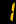# Junior Secondary Curriculum

## Number and Number Systems

• Directed Numbers and the Number Line
• Numerical Estimation
• Approximation and Errors
• Rational and Irrational Numbers

## Comparing Quantities

• Using Percentages
• Rate and Ratio

## Observing Patterns and Expressing Generality

• Formulating Problems with Algebraic Language
• Manipulations of Simple Polynomials
• Law of Integral Indices
• Factorization of Simple Polynomials

## Algebraic Relations and Functions

• Linear Equations in One Unknown
• Linear Equations in Two Unknowns
• Identities
• Formulas
• Linear Inequalities in One Unknown

## Measures in 2D and 3D Figures

• Estimation in Measurement
• Simple Idea of Areas and Volumes
• More about Areas and Volumes

## Learning Geometry Through an Intuitive Approach

• Introduction to Geometry
• Transformation and Symmetry
• Congruence and Similarity
• Angles related with Lines and Rectilinear Figures

## Learning Geometry through a Deductive Approach

• Simple Introduction to Deductive Geometry
• Pythagoras’ Theorem

## Learning Geometry through a Analytic Approach

• Introduction to Coordinates
• Coordinate Geometry of Straight Lines
• Trigonometric Ratios and Using Trigonometry

## Organization and Representation of data

• Introduction to Varios Stages of Statistics
• Construction and Interpretation of Simple Diagrams and Graphs

## Analysis and Interpretation of data

• Measures of Central Tendency

## Probabilty

• Simple Idea of Probability